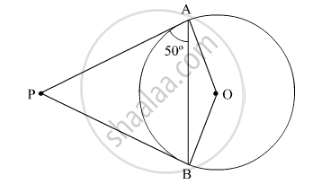# From an external point P, tangents PA and PB are drawn to a circle with centre O. If ∠PAB = 50°, then find ∠AOB. - Mathematics

From an external point P, tangents PA and PB are drawn to a circle with centre O. If ∠PAB = 50°, then find ∠AOB.

#### SolutionIt is given that PA and PB are tangents to the given circle.

PAO=90°      (Radius is perpendicular to the tangent at the point of contact.)

Now

PAB=50°          (Given)

OAB=PAOPAB=90°50°=40°

In ∆OAB,

OB = OA    (Radii of the circle)

OAB=OBA=40°           (Angles opposite to equal sides are equal.)

Now

AOB+OAB+OBA=180°       (Angle sum property)

AOB=180°40°40°=100°

Concept: Number of Tangents from a Point on a Circle
Is there an error in this question or solution?
2015-2016 (March) Delhi Set 1

Share